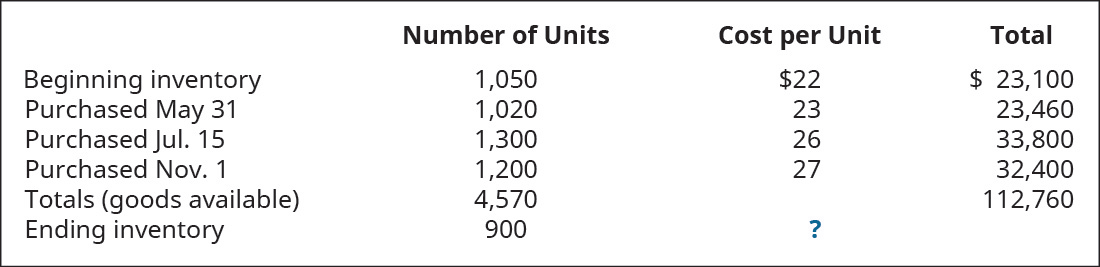# Trini Company had the following transactions for the month. Calculate the ending inventory dollar value for each of the following cost allocation methods, using periodic inventory updating. Provide your calculations. A. first-in, first-out (FIFO) B. last-in, first-out (LIFO) C. weighted average (AVG)FindFindarrow_forward

### Principles of Accounting Volume 1

19th Edition
OpenStax
Publisher: OpenStax College
ISBN: 9781947172685

#### Solutions

Chapter
SectionFindFindarrow_forward

### Principles of Accounting Volume 1

19th Edition
OpenStax
Publisher: OpenStax College
ISBN: 9781947172685
Chapter 10, Problem 2PA
Textbook Problem
9 views

## Trini Company had the following transactions for the month.Calculate the ending inventory dollar value for each of the following cost allocation methods, using periodic inventory updating. Provide your calculations.A. first-in, first-out (FIFO)B. last-in, first-out (LIFO)C. weighted average (AVG)

To determine

(a)

Concept introduction:

FIFO (First in first out): In FIFO Method, company issues that material first, which is purchased earliest. This method provides highest gross margin in case of inflation.

LIFO (Last in first out): In LIFO Method,company issues first that material which comes in last in inventory. This method provides least gross margin in case of inflation.

Weighted average method: In this method, we issue material on random basis, so every type of goods has equal probability.

To calculate:

Value of inventory as per FIFO method.

### Explanation of Solution

Inventory as per FIFO method= No. of units × cost per unit(from last)

&ems...

To determine

(b)

Concept introduction:

LIFO (Last in first out): In LIFO Method,company issues first that material which comes in last in inventory. This method provides least gross margin in case of inflation.

To calculate:

Value of inventory as per LIFO method.

To determine

(c)

Concept introduction:

Weighted average method: In this method, material is issued on random basis.So, every type of goods has equal probability.

To calculate:

Value of inventory as per weighted average method.

### Still sussing out bartleby?

Check out a sample textbook solution.

See a sample solution

#### The Solution to Your Study Problems

Bartleby provides explanations to thousands of textbook problems written by our experts, many with advanced degrees!

Get Started

Find more solutions based on key concepts
All sales, for cash or on credit, can be recorded in the general journal.

College Accounting, Chapters 1-27 (New in Accounting from Heintz and Parry)

Describe the role of prices in market economics.

Principles of Economics (MindTap Course List)

MIRR Project X costs 1,000, and its cash flows arc the same in Years 1 through 10. Its IRR is 12%, and its WACC...

Fundamentals of Financial Management, Concise Edition (with Thomson ONE - Business School Edition, 1 term (6 months) Printed Access Card) (MindTap Course List)